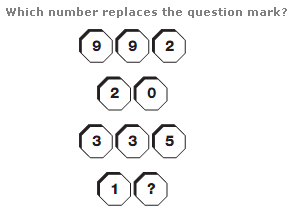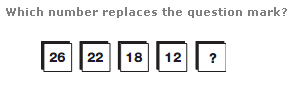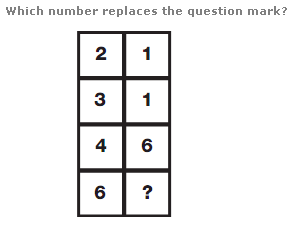# Puzzles - Number puzzles

### Exercise :: Number puzzlesAnswer : 1 Explanation : Taking the top 3 numbers, add them together, and write the 2 digit result in the spaces below. Repeat this pattern for the next set of numbers.Answer : 6 Explanation : Working from left to right, numbers represent the reverse alphabetical values of the vowels in the alphabet.Answer : 6 Explanation : Working from top to bottom, and reading each pair of boxes as a 2 digit number, add 10 to the first number to give the next one down, then add 15, and 20 to complete the sequence.